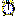#Iteration

An iteration model element makes its parent submodel an iterative submodel, whose contents should be evaluated repeatedly until a finishing condition is met.

## Rules

The iteration symbol contains the condition that marks the successful convergence of the iteration. On each time step of the model, after the compartment values have been adjusted, the values of all variables are calculated as normal. Then if the alarm symbol's equation evaluates to "false", the variables in its submodel are calculated again, and the test repeated, until it evaluates to "true" at which point the loop exits. See iterative submodel for a full description with examples, but a simple case of evaluation by successive approximation could be implemented as follows.

An influence arrow coming from the alarm symbol can be used as an argument to the function iterations( ). This function returns the number of iterations made so far. This function can be used to set the initial value (also called the guess) for the loop, i.e. when the number of iterations so far is equal to zero. This can also be set if the value of the alarm is "true" since this is how it is left at the end of the previous time step. If the number of iterations so far is one or more, or if the value of the alarm is "false", then the result of the last calculation should be used. Since the last calculation depends on the result calculated from the guess, a circular loop of influences is present. Normally, Simile would reject this loop at build time, but setting a property of the influence arrow: "Use values made in same time step" to true, allows the loop to be processed. Influence arrows with this property set are drawn with a dashed line. To set this property for an influence arrow, double-click on it to invoke the property dialogue box.

Note that if the value of the alarm symbol is used directly to decide if the initial guess should be used, then its influence should also be dashed, as the value of the alarm symbol is set by a test on the result. This is not necessary if using the "iterations()" function, which does not check for circularity involving its argument.

• The expression in the equation dialogue box for an iteration element is a Boolean expression: that is, it returns the value "true" or "false". This normally takes the form of some sort of comparison, using the conditional operators such as >, <, >= etc, combined with logical operators such as "and" and "or".